Hybrid Approach for Placement of Type-III Multiple DGs in Distribution Network | OMICS International
Journal of Electrical & Electronic Systems

# Hybrid Approach for Placement of Type-III Multiple DGs in Distribution Network

Kansal S1*, Kumar V2and Tyagi B3

1Department of Electrical Engineering, Baba Hira Singh Bhattal Institute of Engineering and Technology, Lehragaga-148031, Punjab, India

2Department of Electrical Engineering, Indian Institute of Technology, Roorkee, India

3EED, Indian Institute technology, Roorkee, India

*Corresponding Author:
Kansal S
Department of Electrical Engineering
Baba Hira Singh Bhattal Institute of Engineering and Technology
Lehragaga-148031, Punjab, India
Tel: +91-94172-50579
E-mail: [email protected]

Received Date: July 22, 2014; Accepted Date: August 21, 2014; Published Date: September 01, 2014

Citation:Kansal S, Kumar V, Tyagi B (2014) Hybrid Approach for Placement of Type-III Multiple DGs in Distribution Network. J Electr Electron Syst 3:130. doi:10.4172/2332-0796.1000130

Copyright: © 2014 Kansal S, et al. This is an open-access article distributed under the terms of the Creative Commons Attribution License, which permits unrestricted use, distribution, and reproduction in any medium, provided the original author and source are credited.

Visit for more related articles at Journal of Electrical & Electronic Systems

#### Abstract

This paper proposes the hybridization of analytical method and heuristic search for the optimal placement of type-III DGs in power distribution network for reduction of power loss. The type-III DG is capable injecting both real and reactive powers. In this approach the locations are determined by the application of PSO while the sizes of DGs are evaluated by using the analytical method which is based on the exact loss formula. The reduction of power distribution losses has been achieved by compensation of active and reactive powers. The improvement in bus voltage profile and the optimal power factor of the DGs have also been considered. The proposed technique has been tested on a 33-bus test system and the results are compared.

#### Keywords

Distributed generation; Particle Swarm Optimization (PSO); Optimal size; Optimal location; Power loss.

#### Introduction

The newly introduced distributed or decentralized generation units connected to local distribution systems are not dispatchable by central operator, but they can have a significant impact on the power flow, stability, voltage profile, reliability, short circuit level and quality of power supply for customers and electricity suppliers. Optimization techniques should be employed for deregulation of power industry, allowing for the best allocation of the DG.

There are many approaches for deciding the optimum sizing and sitting of distributed generation units in distribution systems. Some of the factors that must be taken into account in the planning process of expanding distribution system with DG are: the number and capacity of DG units, best locations and technology, the network connection, capacity of existing system, protection schemes, among others. Different methodologies and tools have been developed to identify optimal places to install DG capacity and its size. These methodologies are based on analytical tools, optimization programs or heuristic techniques. Most of them find the optimal allocation and size of single DG in order to reduce losses and improve voltage profiles with various techniques [1-3] considered. Others include the placement of multiple DGs with artificial intelligence-based optimization methods and a few go with analytical approach.

In , GA based technique along with Optimal Power Flow (OPF) calculations were used to determine the optimum size and location of DG units installed to the system in order.

To minimize the cost of active and reactive power generation. In , a GA based method was also proposed to find the optimal placement of DG in the compensated network for restoration the system caused by CLPU condition and to conserve load diversity for reduction in losses, improvement in voltage regulation. In , authors proposed a Tabu Search (TS) based method to find the optimal solution of their problem. In , the objective was to minimize a multi-objective performance index function using GA. The indices were reflecting the effect of DG insertion on the real and reactive power losses of the system, the voltage profile, and the distribution line loading with different load models. In , an analytical method to determine the optimum location–size pair of a DG unit was proposed in order to minimize only the line losses of the power system. In , DG units were placed at the most sensitive buses to voltage collapse. The units had the same capacity and were placed one by one.

In , a Particle Swarm Optimization (PSO) algorithm was introduced to determine the optimal size and location of DG and Capacitor unit to compensate the active and reactive powers of the distribution system. The evaluation of optimal power factor and improvement in voltage profile has also been considered in this work.

Most of the researches placed DG units with unity power factor. An analytical approach based on exact loss formula was presented to find the optimal size and location of DG to minimize the real power loss , although the results violate the voltage constraint. Recently, another fast analytical approach to find the optimal size of DG at optimal power factor to minimize the power losses however only type III has been exploited .

The present work develops the comprehensive formula by extending the analytical expression presented in  to find the optimal size of multiple DGs supplying real and reactive power and a search to identify best locations and optimal power factor to achieve the objective by compensating the active and reactive powers. Besides, voltage profile enhancement is also examined and the results of the proposed hybrid approach are verified with existing technique.

#### Mathematical Background

The total power loss has been formulated as is given by (1).This formula is popularly referred as “Exact Loss” formula .

Where,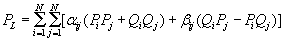(1)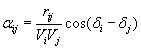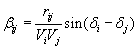and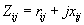is the ijth element of [Zbus] matrix

N-Total number of buses.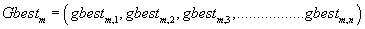(2)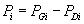(3)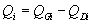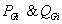are generated active and reactive powers at ith bus respectively;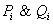are active and reactive power injections at ithbus respectively;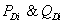are the active and reactive loads at ith bus respectively.

Sizing of multiple DGs

The real power loss formula (1) is used to determine the sizes of multiple DGs at respective buses to minimize the power loss.

Considering,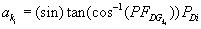The reactive power output of DG, where n is the number of DGs and is the bus number of ith DG is given by(4)

In which (+) sign is for injecting reactive power and (-) sign is consuming reactive power by DG.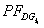is the power factor of DG at kith bus of ith DG, which equal to power factor of system load.

The active and reactive power injected at bus kith, where DG is located, are given by (5) and (6), respectively,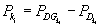(5)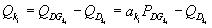(6)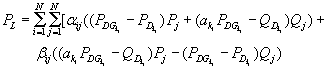Differentiate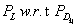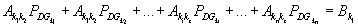Differentiate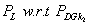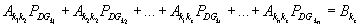Similarly Differentiate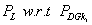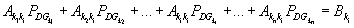Where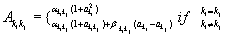(7)

There will be n equations with n variables, which can be solved as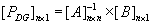(8)

Where,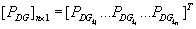(9)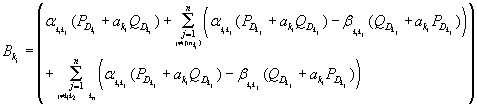(10)

Equation (9) and (4) provides the sizes of multiple DGs at respective bus for the losses to be minimum.

#### Optimal power factor of DGs

With obtained optimal sizes of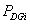and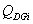the power factor of the DGs is considered as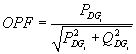(11)

#### Optimal locations of DGs

For single DG placement, it is possible to calculate DG size and to evaluate the loss at every bus by analytical approach. But when it comes to determine combination of N buses in the same network for n DGs, the number of combinations will be NCn, so a search technique or a heuristic method needs to be implemented to find the optimal locations. The optimal locations for the placement of multiple DGs are determined by using PSO technique taking the location and optimal power factor of each DG as the variable.

#### Problem Formulation

Objective function

The main objective is to minimize the total power loss as given in (1) while meeting the following constraints.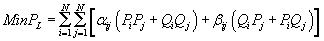(12)

The network power flow equation must be satisfied,

The sizing and locations are considered at point load only,

The voltage at every bus in the network should be within the acceptable range (Utility’s standard ANSI Std. C84.1-1989) i.e., within permissible limit (±5%) ,

Vmin ≤ Vi ≤ Vmax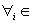{buses of the network}

Current in a feeder or conductor, must be well within the maximum thermal capacity of the conductor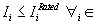{Branches of the network}

Here,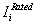is current permissible for branch i within safe limit of temperature.

Distributed Generation is defined as the generation of electricity by facilities that are sufficiently smaller than the central generating plants so to allow interconnection at nearly any point in a power system. There is no defined limit on the amount of generation through DG. For example, in  and  the maximum DG installed capacity limits have been considered as 30% and 50% respectively. Hence, here the total installed capacity of DG in the network has been limited to less than 30% of substation rated capacity plus line losses to maintain the concept of DG against centralized generation similar to .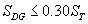(13)

Where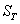is the rating of the transformer

Computational procedure

The proposed approach has been used for determining the optimal placement of multiple DGs, and is given step by step in the following subsections. The backward sweep and forward sweep method of load flow  is used for radial network solution.

Particle swarm optimization technique.

Particle Swarm Optimization (PSO) is a population-based optimization technique which provides a population-based search procedure in which individuals called particles change their position (state) with time. In a PSO system, particles fly around in a n-dimensional search space. During flight, each particle adjusts its position according to its own experience (This value is called pbest), and according to the experience of a neighboring particle (This value is called gbest), made use of the best position encountered by itself and its neighbor .

Mathematically, the position of particle in an n-dimensional vector is represented as: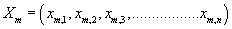(14)

The velocity of this particle is also an n-dimensional vector,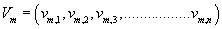(14)

Alternatively, the best position related to the lowest value (for objective minimization) of the objective function for each particle is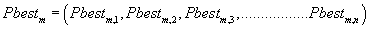and the global best position among all the particles or best pbest is denoted as:During the iteration procedure, the velocity and position of the particles are updated. The population size of swarms and iterations are fixed i.e. the PSO parameters, population size of swarms and iterations are taken 50 and 60 respectively. The population of mth particles Xm (consisting of location and power factor of DG) as well as their velocity Vm in the search space is initialized as given in (13-14). The appropriate values for weights ωmin and ωmax are 0.4 and 0.9  are set respectively.

The present work extends the analytical expressions presented in  to find the optimal sizes of multiple DGs and optimal locations with PSO technique. The number of DGs (n=1, 2, 3…) are considered to minimize the power loss. The computational procedure to find the optimal sizes at locations of multiple DGs is described below.

Step 1: Input line and bus data, and constraints.

Step 2: Enter the number of DG units.

Step 3: Run the load flow for the base case and calculate the losses using (1).

Step 4: Find the size of DGs for each bus using (9) and (4).

Step 5: Initialize random values into particles which correspond to bus numbers or locations of DGs of the given network. Set the iteration counter k=0.

Step 6: Take the first particle as the locations of DGs, there are number of possible locations as the number of particles

Step 7:Find the fitness value for the every selected location for DGs using (1).

Step 8: Update the weight, velocity and position of each particle.

Step 9: if the iteration number reaches the maximum limit, go to step 10. Otherwise, set the iteration index k=k+1, and go back to step 5.

Step 10: Print out the optimal solution to the target problem. The best position includes the optimal locations and sizes of DGs and representing the corresponding minimum total real power loss.

#### Numerical results

Test system

The proposed methodology is tested on test system contains 33 buses and 32 branches as shown in Figure 1. It is a radial system with a total load of 3.72 MW and 2.3 MVAR  with Beaver conductor. The base voltage for the test system is 12.66 kV. An analytical software tool has been developed in MATLAB environment for the proposed approach to run load flow, calculate losses. The optimal sizes of DGs at optimal locations are determined to achieve the objective. The maximum number of DG units installed considered to be three and the total capacity of the DG units is also assumed to equal to the total load plus line losses. The sizes of DGs are set less than 30% of substation rated capacity plus line losses.

DG placement at optimal power factor

Table 1 shows the placement of multiple DGs supplying both real and reactive powers. The maximum sizes of DGs installed are 30% of the rated capacity of substation plus line losses. The results of the base case and three cases with DG numbers ranging from one to three are determined. The result includes the optimal sizes, optimal locations and optimal power factor of DGs with respect to the total loss. The power factor of the DG must be opposite to the power factor of bus load. The 33-bus system has a lagging power factor load; hence the power factor of DG must be leading. Consequently, the net total of both active and reactive power of that bus where the DG is placed will decrease.

The loss reduction and schedule of installed DGs are presented in the Table 1. For single DG, the loss reduction by the hybrid approach is 64.18% and by two DGs and three DGs the loss reductions are 79.84% and 80.62% respectively. It is observed that as the number of DGs is increased, the reduction in line losses is more effective.

Cases Installed DG Schedule Ploss (kW) Loss Reduction (%)
No DG         211 0
I DG Location 30     75.6 64.18
Size (kVA) 1749
OPF 0.86
Locations 14 30
2 DGs Size (kVA) 705 1076   42.53 79.84
OPF 0.95 0.77
Locations 14 30 32
3 DGs Size (kVA) 706 663 430 40.88 80.62
OPF 0.95 0.67 0..89

Table 1: Type-III DG Placement for 33-Bus System.

Comparative study

Although the proposed hybrid approach proved its robustness in solving the test case, additional case of DG without technical size constraints was adopted on 33-bus radial distributed feeder system for comparison purpose. Therefore, the results of the proposed hybrid approach was compared with the solutions obtained based on the Improved analytical method . Table 2 summarizes the optimal solutions achieved by these methods.

Cases Technique Installed DG Schedule Ploss (kW)
No DG           211
I DG Unit Improved Analytical Location 6     67.9
Size (kVA) 3107
OPF 0.82
Hybrid Approach Location 6     67.95
Size (kVA) 3028
OPF 0.82
2 DG units Improved Analytical  Locations 6 30   44.39
Size (kVA) 2195 1098
OPF 0.82 0.82
Hybrid Approach Locations 13 30   28.55
Size (kVA) 828 1114
OPF 0.91 0.73
3 DG units Improved Analytical  Locations 6 30 14 22.29
Size (kVA) 1098 1098 768
OPF 0.82 0.82 0.82
Hybrid Approach Locations 13 24 30 11.76
Size (kVA) 782 1069 1016
OPF 0.91 0.9 0.71

Table 2: Comparison of DG placement results for the 33-bus system.

Observing Table 2, the optimal placement of the single DG, bus location, optimal power factor and reduction in line losses were nearly identical, with reduction in size of DG. For two DG placement the reduction in line loss was 28.55 kW by the hybrid approach as compared to 44.39 kW by Improved Analytical approach .

As seen in the Improved Analytical approach the DGs are placed one by one with fixed OPF, whereas in the proposed hybrid approach the numbers of DGs are placed simultaneously with their OPFs.

For placement of three DGs, the reduction in losses was 11.76 kW as compared to 22.29 kW of Fast Analytical approach. However, if the optimal DG size in the analytical methods were rounded off to the closest practical one, the accuracy of the results would be affected. The proposed hybrid approach avoids this limitation and accuracy of the results is guaranteed.

Voltage profile

Figures 2-4 indicate the minimum and maximum voltages before and after the placement of 1 DG, 2 DGs and 3 DGs for 33-bus test system.

It is seen that in all the cases the voltage profile improves, when the number of DG units installed in the system is increased, while satisfy all the current and voltage constraints.

#### Conclusion

In the proposed hybrid approach, the sizes of DGs are evaluated by analytical approach and the locations are determined by the application of PSO approach. This paper has presented the allocation of multiple DGs capable of injecting both real and reactive powers for active and reactive power compensation to minimize the line losses in the primary distribution networks. The number of DG units with appropriate sizes at locations can reduce the losses to a considerable amount. The optimal power factor which results minimum power loss has also been determined. The proposed approach of optimal placement of multiple DGs not only reduces the line losses but also minimize the sizes of DGs with satisfaction of the permissible voltage limits. In the age of integrated grid, the placement and analysis of multiple DGs give guidance for optimal operation of power system.

#### References

Select your language of interest to view the total content in your interested language

### Article Usage

• Total views: 12333
• [From(publication date):
December-2014 - Dec 08, 2019]
• Breakdown by view type
• HTML page views : 8497Can't read the image? click here to refresh